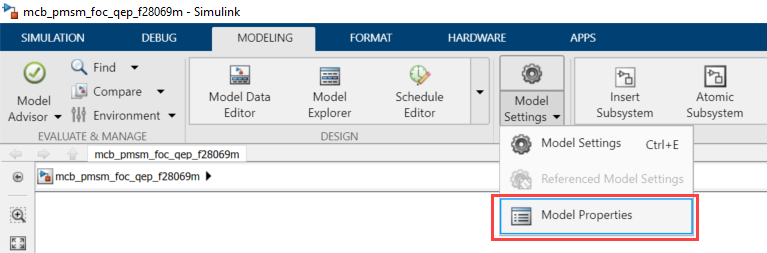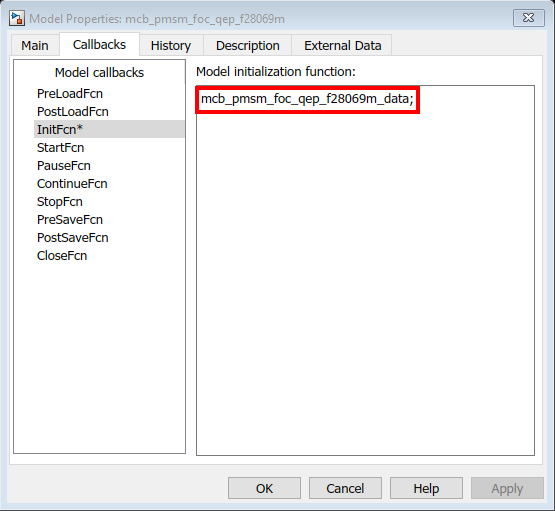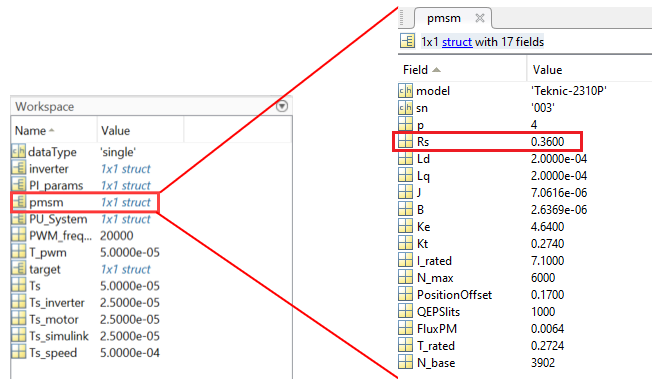Main Content

## Estimate Control Gains from Motor Parameters

Perform control parameter tuning for the speed and the torque control loops that are part of the Field-Oriented Control (FOC) algorithm. Motor Control Blockset™ provides you with multiple methods to compute the control loop gains from the system or block transfer functions that are available for the motors, inverter, and controller:

• Use the Field Oriented Control Autotuner block.

• Use Simulink® Control Design™.

• Use the model initialization script.### Field-Oriented Control Autotuner

The Field-Oriented Control Autotuner block of Motor Control Blockset enables you to automatically tune the PID control loops in your Field-Oriented Control (FOC) application in real time. You can automatically tune the PID controllers associated with the following loops (for more details, see How to Use Field Oriented Control Autotuner Block):

• Direct-axis (d-axis) current loop

• Quadrature-axis (q-axis) current loop

• Speed loop

For each loop that the block tunes, the Field-Oriented Control Autotuner block performs the autotuning experiment in a closed-loop manner without using a parametric model associated with that loop. The block enables you to specify the order in which the block tunes the control loops. When the tuning experiment runs for one loop, the block has no effect on the other loops. For more details about FOC autotuner, see Field Oriented Control Autotuner and Tune PI Controllers Using Field Oriented Control Autotuner.

### Simulink Control Design

Simulink Control Design enables you to design and analyze the control systems modeled in Simulink. You can automatically tune the arbitrary SISO and MIMO control architectures, including the PID controllers. You can deploy PID autotuning to the embedded software to automatically compute the PID gains in real time.

You can find the operating points and compute the exact linearizations of the Simulink models at different operating conditions. Simulink Control Design provides tools that let you compute the simulation-based frequency responses without modifying your model. For details, see https://www.mathworks.com/help/slcontrol/index.html.

### Model Initialization Script

This section explains how the Motor Control Blockset examples estimate the control gains needed to implement field-oriented control. For example, for a PMSM that is connected to a quadrature encoder, these steps describe the procedure to compute the control loop gain values from the system details by using the initialization script:

1. Open the initialization script (`.m`) file of the example in MATLAB®. To find the associated script file name:

1. Select Modeling > Model Settings > Model Properties to open the model properties dialog box.2. In the Model Properties dialog box, navigate to the Callbacks tab > InitFcn to find the name of the script file that Simulink opens before running the example.2. This figure shows an example of the initialization script (`.m`) file.3. Use the Workspace to edit the control variables values. For example, to update Stator resistance (`Rs`), use the variable `pmsm` to add the parameter value to the `Rs` field.4. The model initialization script associated with a target model calls these functions and sets up the workspace with the necessary variables.

Model Initialization ScriptFunction Called By Model Initialization ScriptDescription
Script associated with a target model

`mcb_SetPMSMMotorParameters`

Input to the function is motor type (for example, BLY171D).

The function populates a structure named `pmsm` in the MATLAB workspace, which is used by the model.

It also computes the permanent magnet flux and rated torque for the selected motor.

You can extend the function by adding an additional switch-case for a new motor.

This function also loads the structure `motorParam`, obtained by running parameter estimation, to the structure `pmsm`. If the structure `motorParam` is not available in the MATLAB workspace, the function loads the default parameters.

`mcb_SetInverterParameters`

Input to the function is inverter type (for example, BoostXL-DRV8305).

The function populates a structure named `inverter` in the MATLAB workspace, which is used by the model.

The function also computes the inverter resistance for the selected inverter.

You can extend the function by adding an additional switch-case for a new inverter.

`mcb_SetProcessorDetails`

Inputs to the function are processor type (for example, F28379D) and the Pulse-Width Modulation (PWM) switching frequency.

The function populates a structure named `target` in the MATLAB workspace, which is used by the model.

The function also computes the PWM counter period that is a parameter for the ePWM block in the target model.

You can extend the function by adding an additional switch-case for a new processor.

`mcb_getBaseSpeed`

Inputs to the function are motor and inverter parameters.

The function computes the base speed for PMSM.

Type ```help mcb_getBaseSpeed``` at the MATLAB command window or see section Obtain Base Speed for more details.

`mcb_SetPUSystem`

Inputs to the function are motor and inverter parameters.

The function sets the base values of the per-unit system for voltage, current, speed, torque, and power.

The function populates a structure named `PU_System` in the MATLAB workspace, which is used by the model.

`mcb.internal.SetControllerParameters`

Inputs to the function are motor and inverter parameters, per-unit system base values, PWM switching time period, sample time for the control system, and sample time for the speed controller.

The function computes the Proportional Integral (PI) parameters (Kp, Ki) for the field-oriented control implementation.

The function populates a structure named `PI_params` in the MATLAB workspace, which is used by the model.

See section Obtain Controller Gains for more details.

`mcb_updateInverterParameters`

Inputs to the function are motor and inverter parameters.

The function updates the inverter parameters based on the selected hardware and motor.

This table explains the useful variables for each control parameter that you can update.

Note

You can try starting MATLAB in the administrator mode on Windows® system, if you are unable to update the model initialization scripts associated with the example models.

Control Parameter CategoryControl Parameter NameMATLAB Workspace Variable
Motor parametersManufacturer’s model number`pmsm.model`
Manufacturer’s serial number`pmsm.sn`
Pole pairs`pmsm.p`
Stator resistance (Ohm)`pmsm.Rs`
d-axis stator winding inductance (Henry)`pmsm.Ld`
q-axis stator winding inductance (Henry)`pmsm.Lq`
Back emf constant (V_line(peak)/krpm)`pmsm.Ke`
Motor Inertia (kg.m2)`pmsm.J`
Friction constant (N.m.s)`pmsm.F`
Permanent Magnet Flux (WB)`pmsm.FluxPM`
Trated`pmsm.T_rated`
Nbase`pmsm.N_base`
Irated`pmsm.I_rated`
Position decodersQEP index and Hall position offset correction`pmsm.PositionOffset`
Quadrature encoder slits per revolution`pmsm.QEPSlits`
Inverter parametersManufacturer’s model number`inverter.model`
Manufacturer’s serial number`inverter.sn`
DC link voltage of the inverter (V)`inverter.V_dc`
Maximum permissible currents by inverter (A)`inverter.I_trip`
On-state resistance of MOSFETs (Ohm)`inverter.Rds_on`
Shunt resistance for current sensing (Ohm)`inverter.Rshunt`
Per-phase board resistance seen by motor (Ohm)`inverter.R_board`
ADC Offsets for current sensor (Ia and Ib)

`inverter.CtSensAOffset`

`inverter.CtSensBOffset`

Maximum limit of automatically calibrated ADC offsets for current sensor (Ia and Ib)`inverter.CtSensOffsetMax`
Minimum limit of automatically calibrated ADC offsets for current sensor (Ia and Ib)`inverter.CtSensOffsetMin`
Enable Auto-calibration for current sense ADCs`inverter.ADCOffsetCalibEnable`
ADC gain factor configured by SPI`inverter.ADCGain`

Type of inverter:

1 — Active high-enabled inverter.

0 — Active low-enabled inverter.

`inverter.EnableLogic`

Type of current sense amplifier:

1 — Non-inverting amplifier

–1 — Inverting amplifier

`inverter.invertingAmp`
Reference voltage for the inverter current sensing circuit (V)`inverter.ISenseVref`

Output voltage of the inverter current sensing circuit corresponding to 1 Ampere current (V/A)

You can compute this parameter using the datasheet values of current shunt resistance (`inverter.Rshunt`) and current sense amplifier gain of the inverter.

`inverter.ISenseVoltPerAmp` = `inverter.Rshunt` ✖ current sense amplifier gain

`inverter.ISenseVoltPerAmp`
Maximum measurable peak-neutral current by the inverter current sensing circuit (A)`inverter.ISenseMax`
ProcessorManufacturer’s model number`target.model`
Manufacturer’s serial number`target.sn`
CPU Frequency`target.CPU_frequency`
PWM frequency`target.PWM_frequency`
PWM counter period`target.PWM_Counter_Period`
Reference voltage for ADC (V)`Target.ADC_Vref`
Maximum count output for 12-bit ADC`Target.ADC_MaxCount`
Baud rate for serial communication`Target.SCI_baud_rate`
Per-Unit SystemBase voltage (V)`PU_System.V_base`
Base current (A)`PU_System.I_base`
Base speed (rpm)`PU_System.N_base`
Base torque (Nm)`PU_System.T_base`
Base power (Watts)`PU_System.P_base`
Data-type for target deviceData-type (Fixed-point Or Floating-point) selection`dataType`
Sample time valuesSwitching frequency for converter`PWM_frequency`
PWM switching time period`T_pwm`
Sample time for current controllers`Ts`
Sample time for speed controller`Ts_speed`
Simulation sample time`Ts_simulink`
Simulation sample time for motor`Ts_motor`
Simulation sample time for inverter`Ts_inverter`
Controller parametersProportional gain for Iq controller`PI_params.Kp_i`
Integral gain for Iq controller`PI_params.Ki_i`
Proportional gain for Id controller`PI_params.Kp_id`
Integral gain for Id controller`PI_params.Ki_id`
Proportional gain for Speed controller`PI_params.Kp_speed`
Integral gain for Speed controller`PI_params.Ki_speed`
Proportional gain for Field weakening controller`PI_params.Kp_fwc`
Integral gain for Field weakening controller`PI_params.Ki_fwc`
Sensor delay parametersCurrent sensor delay`Delays.Current_Sensor`
Speed sensor delay`Delays.Speed_Sensor`
Delay for low-pass speed filter`Delays.Speed_Filter`
Controller delay parametersDamping factor (ζ) of the current control loop`Delays.OM_damping_factor`
Symmetrical optimum factor of the speed control loop`Delays.SO_factor_speed`

Note

For the predefined processors and drivers, the model initialization script uses the default values.

The model initialization script uses these functions for performing the computations:

Control Parameter CategoryFunctionFunctionality
Base speed of the motor`mcb_getBaseSpeed`

Calculates the base speed of PMSM at the rated voltage and rated load.

For details, type ```help mcb_getBaseSpeed``` at the MATLAB command prompt or see section Obtain Base Speed.

Motor characteristics for the given motor and inverter`mcb_getCharacteristics`

Obtain these characteristics of the motor.

• Torque as opposed to speed characteristics

• Power as opposed to speed characteristics

• Iq as opposed to speed and Id as opposed to speed characteristics

For details, type ```help mcb_getCharacteristics``` at the MATLAB command prompt.

Control algorithm parameters`mcb.internal.SetControllerParameters`

Compute the gains for these PI controllers:

• Current (torque) control loop gains (Kp, Ki) for currents Id and Iq

• Speed control loop gains (Kp, Ki)

• Field weakening control gains (Kp, Ki)

For details, see section Obtain Controller Gains.

Control analysis for the motor and inverter you are using`mcb_getControlAnalysis`

Performs frequency domain analysis for the computed gains of PI controllers used in the field-oriented motor control system.

Note

This feature requires Control System Toolbox™.

For details, type ```help mcb_getControlAnalysis``` at the MATLAB command prompt.

#### Obtain Base Speed

The function `mcb_getBaseSpeed` computes the base speed of the PMSM at the given supply voltage. Base speed is the maximum motor speed at the rated voltage and rated load, outside the field-weakening region.

When you call this function (for example, ```base_speed = mcb_getBaseSpeed(pmsm,inverter)```), it returns the base speed (in rpm) for the given combination of PMSM and inverter. The function accepts the following inputs:

• PMSM parameter structure.

• Inverter parameter structure.

These equations describe the computations that the function performs:

The inverter voltage constraint is defined by computing the d-axis and q-axis voltages:

The current limit circle defines the current constraint which can be considered as:

In the preceding equation, ${i}_{d}$ is zero for surface PMSMs. For interior PMSMs, values of ${i}_{d}$ and ${i}_{q}$ corresponding to MTPA are considered.

Using the preceding relationships, we can compute the base speed as:

where:

• ${\omega }_{e}$ is the electrical speed corresponding to frequency of stator voltages (Radians/ sec).

• ${\omega }_{base}$ is the mechanical base speed of the motor (Radians/ sec).

• ${i}_{d}$ is the d-axis current (Amperes).

• ${i}_{q}$ is the q-axis current (Amperes).

• ${v}_{do}$ is the d-axis voltage when ${i}_{d}$ is zero (Volts).

• ${v}_{qo}$ is the q-axis voltage when ${i}_{q}$ is zero (Volts).

• ${L}_{d}$ is the d-axis winding inductance (Henry).

• ${L}_{q}$ is the q-axis winding inductance (Henry).

• ${R}_{s}$ is the stator phase winding resistance (Ohms).

• ${\lambda }_{pm}$ is the permanent magnet flux linkage (Weber).

• ${v}_{d}$ is the d-axis voltage (Volts).

• ${v}_{q}$ is the q-axis voltage (Volts).

• ${v}_{max}$ is the maximum fundamental line to neutral voltage (peak) supplied to the motor (Volts).

• ${v}_{dc}$ is the dc voltage supplied to the inverter (Volts).

• ${i}_{max}$ is the maximum phase current (peak) of the motor (Amperes).

• $p$ is the number of motor pole pairs.

#### Obtain Motor Characteristics

The function `mcb_getCharacteristics` calculates the torque and speed characteristics of the motor, which helps you to develop the control algorithm for the motor.

The function returns these characteristics for the given PMSM:

• Torque as opposed to Speed

• Power as opposed to Speed

• Iq as opposed to Speed

• Id as opposed to Speed#### Obtain Controller Gains

The function `mcb.internal.SetControllerParameters` computes the gains for the PI controllers used in the field-oriented motor control systems.

You can use this command to call the function `mcb.internal.SetControllerParameters`:

`PI_params = mcb.internal.SetControllerParameters(pmsm,inverter,PU_System,T_pwm,Ts,Ts_speed);`

The function returns the gains of these PI controllers used in the FOC algorithm:

• Direct-axis (d-axis) current loop

• Quadrature-axis (q-axis) current loop

• Speed loop

• Field-weakening control loop

The function accepts these inputs:

• `pmsm object`

• `inverter object`

• `PU system params`

• `T_pwm`

• `Ts_control`

• `Ts_speed`

The function does not plot any characteristic.

The design of compensators depends on the classical frequency response analysis applied to the motor control systems. We used the Modulus Optimum (MO) based design for the current controllers and the Symmetrical Optimum (SO) based design for the speed controller.

The function automatically computes the other required parameters (for example, delays, damping factor) based on the input arguments.

You can modify the default system responses by an optional input to the function that specifies the system delays, damping factor, and symmetrical optimum factor:

`PI_params = mcb.internal.SetControllerParameters(pmsm,inverter,PU_System,T_pwm,Ts,Ts_speed,Delays);`

Damping factor (ζ) defines the dynamic behavior of the standard form of a second-order system, where `0` < ζ < `1` . An underdamped system gets close to the final value more quickly than a critically damped or an overdamped system. Among the systems that respond without oscillations, a critically damped system shows the quickest response. An overdamped system is always slow in responding to any inputs. This parameter has a default value of $\frac{1}{\sqrt{2}}$.

Symmetrical optimum factor (a) defines the placement of the cross-over frequency at the geometric mean of the two corner frequencies, to obtain maximum phase margin that results in optimum damping of the speed loop, where a > `1` . This parameter has a default value of `1.2`.

This example explains how to customize the parameters:

```% Sensor Delays Delays.Current_Sensor = 2*Ts; %Current Sensor Delay Delays.Speed_Sensor = Ts; %Speed Sensor Delay Delays.Speed_Filter = 20e-3; %Delay for Speed filter (LPF) % Controller Delays Delays.OM_damping_factor = 1/sqrt(2); %Damping factor for current control loop Delays.SO_factor_speed = 1.5; %Symmetrical optimum factor 1 < x < 20 % Controller design PI_params = mcb.internal.SetControllerParameters(pmsm,inverter,PU_System,T_pwm,Ts,Ts_speed,Delays);```

#### Perform Control Analysis

The function `mcb_getControlAnalysis` performs the basic control analysis of the PMSM FOC current control system. The function performs frequency domain analysis for the computed PI controller gains used in the field-oriented motor control systems.

Note

This function requires the Control System Toolbox.

When you call this function (for example, `mcb_getControlAnalysis(pmsm,inverter,PU_System,PI_params,Ts,Ts_speed)`), it performs the following functions for the current control loop or subsystem:

• Transfer function for the closed-loop current control system

• Root locus

• Bode diagram

• Stability margins (PM & GM)

• Step response

• PZ map

The function plots the corresponding plots:Ogata, K. (2010). Modern control engineering. Prentice hall.

 Leonhard, W. (2001). Control of electrical drives. Springer Science & Business Media. pp. 86.

Get ebook